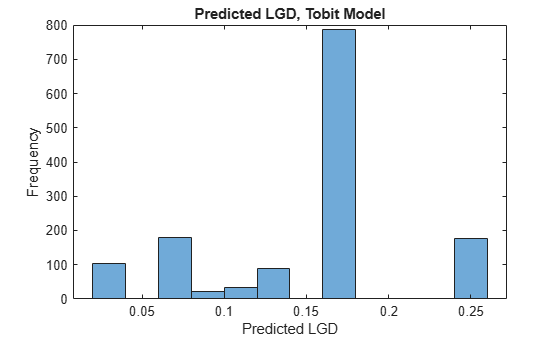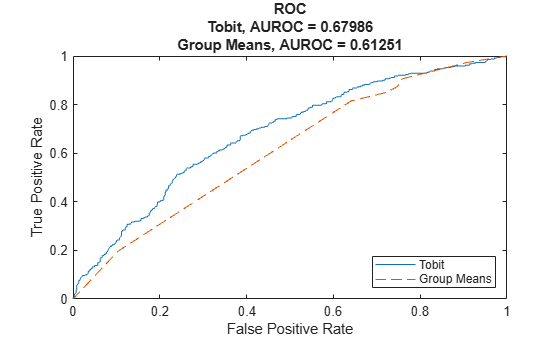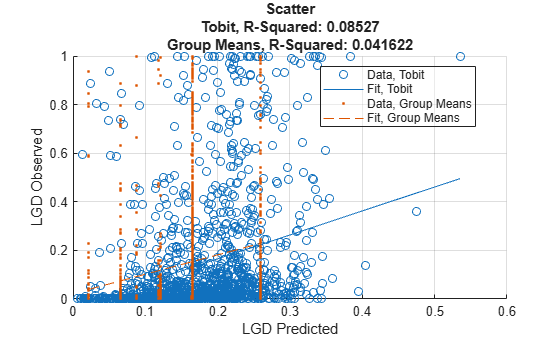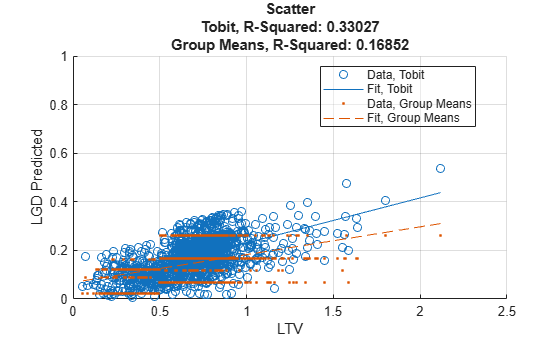# Compare Tobit LGD Model to Benchmark Model

This example shows how to compare a `Tobit` model for loss given default (LGD) against a benchmark model.

```load LGDData.mat disp(head(data))```
``` LTV Age Type LGD _______ _______ ___________ _________ 0.89101 0.39716 residential 0.032659 0.70176 2.0939 residential 0.43564 0.72078 2.7948 residential 0.0064766 0.37013 1.237 residential 0.007947 0.36492 2.5818 residential 0 0.796 1.5957 residential 0.14572 0.60203 1.1599 residential 0.025688 0.92005 0.50253 investment 0.063182 ```

Split the data into training and test sets.

```NumObs = height(data); rng('default'); % For reproducibility c = cvpartition(NumObs,'HoldOut',0.4); TrainingInd = training(c); TestInd = test(c);```

### Fit Tobit Model

Fit a `Tobit` LGD model with training data. By default, the last column of the data is used as a response variable and all other columns are used as predictor variables.

```lgdModel = fitLGDModel(data(TrainingInd,:),'tobit'); disp(lgdModel)```
``` Tobit with properties: CensoringSide: "both" LeftLimit: 0 RightLimit: 1 ModelID: "Tobit" Description: "" UnderlyingModel: [1x1 risk.internal.credit.TobitModel] PredictorVars: ["LTV" "Age" "Type"] ResponseVar: "LGD" ```
`disp(lgdModel.UnderlyingModel)`
```Tobit regression model: LGD = max(0,min(Y*,1)) Y* ~ 1 + LTV + Age + Type Estimated coefficients: Estimate SE tStat pValue _________ _________ _______ __________ (Intercept) 0.058257 0.027279 2.1356 0.032828 LTV 0.20126 0.031383 6.4129 1.7592e-10 Age -0.095407 0.0072435 -13.171 0 Type_investment 0.10208 0.018054 5.6544 1.7785e-08 (Sigma) 0.29288 0.0057071 51.318 0 Number of observations: 2093 Number of left-censored observations: 547 Number of uncensored observations: 1521 Number of right-censored observations: 25 Log-likelihood: -698.383 ```

You can now use this model for prediction or validation. For example, use `predict` to predict LGD on test data and visualize the predictions with a histogram.

```lgdPredTobit = predict(lgdModel,data(TestInd,:)); histogram(lgdPredTobit) title('Predicted LGD, Tobit Model') xlabel('Predicted LGD') ylabel('Frequency')```### Create Benchmark Model

In this example, the benchmark model is a lookup table model that segments the data into groups and assigns the mean LGD of the group to all group members. In practice, this common benchmarking approach is easy to understand and use.

The groups in this example are defined using the three predictors. `LTV` is discretized into low and high levels. `Age` is discretized into young and old loans. `Type` already has two levels, namely, residential and investment. The groups are all the combinations of these values (for example, low LTV, young loan, residential, and so on). The number of levels and the specific cutoff points are only for illustration purposes. The benchmark model uses the same predictors as the `Tobit` model in this example, but you can use other variables to define the groups. In fact, the benchmark model could be a black-box model as long as the predicted LGD values are available for the same customers as in this data set.

```% Add the discretized variables as new colums in the table. % Discretize the LTV. LTVEdges = [0 0.5 max(data.LTV)]; data.LTVDiscretized = discretize(data.LTV,LTVEdges,'Categorical',{'low','high'}); % Discretize the Age. AgeEdges = [0 2 max(data.Age)]; data.AgeDiscretized = discretize(data.Age,AgeEdges,'Categorical',{'young','old'}); % Type is already a categorical variable with two levels.```

Finding the group means on the training data is effectively the fitting of the model. Note that the group counts are small for some groups. Adding many groups comes with reduced group counts for some groups and more unstable estimates.

```% Find the group means on training data. gs = groupsummary(data(TrainingInd,:),{'LTVDiscretized','AgeDiscretized','Type'},'mean','LGD'); disp(gs)```
``` LTVDiscretized AgeDiscretized Type GroupCount mean_LGD ______________ ______________ ___________ __________ ________ low young residential 163 0.12166 low young investment 26 0.087331 low old residential 175 0.021776 low old investment 23 0.16379 high young residential 1134 0.16489 high young investment 257 0.25977 high old residential 265 0.066068 high old investment 50 0.11779 ```

To predict an LGD for a new observation, you need to find its group and then assign the group mean as the predicted LGD. Use the `findgroups` function, which takes the discretized variables as input. For a completely new data point, the `LTV` and `Age` information needs to be discretized first by using the `discretize` function before you use the `findgroups` function.

```LGDGroup = findgroups(data(TestInd,{'LTVDiscretized' 'AgeDiscretized' 'Type'})); lgdPredMeansTest = gs.mean_LGD(LGDGroup);```

There are eight unique values in the predictions, as expected, one for each group.

`disp(unique(lgdPredMeansTest))`
``` 0.0218 0.0661 0.0873 0.1178 0.1217 0.1638 0.1649 0.2598 ```

The histogram of the predictions also shows the discrete nature of the model.

```histogram(lgdPredMeansTest) title('Predicted LGD, Tobit Model') xlabel('Predicted LGD') ylabel('Frequency')```To have all the predictions available for both training and test sets to make comparisons, add a column with LGD predictions for the entire data set.

```LGDGroup = findgroups(data(:,{'LTVDiscretized' 'AgeDiscretized' 'Type'})); data.lgdPredMeans = gs.mean_LGD(LGDGroup);```

### Compare Performance

Compare the performance of the Tobit model and the benchmark model using the validation functions in the `Tobit` model.

Start with the area under the receiver operating characteristic (ROC) curve, or AUROC metric, using `modelDiscrimination`.

```DataSetChoice ="Testing"; if DataSetChoice=="Training" Ind = TrainingInd; else Ind = TestInd; end DiscMeasure = modelDiscrimination(lgdModel,data(Ind,:),'ShowDetails',true,'ReferenceLGD',data.lgdPredMeans(Ind),'ReferenceID','Group Means')```
```DiscMeasure=2×3 table AUROC Segment SegmentCount _______ __________ ____________ Tobit 0.67986 "all_data" 1394 Group Means 0.61251 "all_data" 1394 ```

Use `modelDiscriminationPlot` to visualize the ROC curve.

`modelDiscriminationPlot(lgdModel,data(Ind,:),'ReferenceLGD',data.lgdPredMeans(Ind),'ReferenceID','Group Means')`Use `modelCalibration` to compute the calibration metrics.

`CalMeasure = modelCalibration(lgdModel,data(Ind,:),'ReferenceLGD',data.lgdPredMeans(Ind),'ReferenceID','Group Means')`
```CalMeasure=2×4 table RSquared RMSE Correlation SampleMeanError ________ _______ ___________ _______________ Tobit 0.08527 0.23712 0.29201 -0.034412 Group Means 0.041622 0.2406 0.20401 -0.0078124 ```

Use `modelCalibrationPlot` to visualize the scatter plot of the observed LGD values against predicted LGD values.

`modelCalibrationPlot(lgdModel,data(Ind,:),'ReferenceLGD',data.lgdPredMeans(Ind),'ReferenceID','Group Means')`Then you can use `modelCalibrationPlot` to visualize the scatter plot of the predicted LGD values against the LTV values.

`modelCalibrationPlot(lgdModel,data(Ind,:),'ReferenceLGD',data.lgdPredMeans(Ind),'ReferenceID','Group Means','XData','LTV','YData','predicted')`